# 19 Results

View
Selected filters:
• GeometryConditional Remix & Share Permitted
CC BY-NC-SA
Rating

This textbook is an introductory coverage of algorithms and data structures with application to graphics and geometry.

Subject:
Mathematics
Geometry
Material Type:
Textbook
Provider:
Global Text Project
Author:
Jurg Nievergelt
Klaus Hinrichs
01/01/2011Conditional Remix & Share Permitted
CC BY-NC-SA
Rating

This task was developed by high school and postsecondary mathematics and design/pre-construction educators, and validated by content experts in the Common Core State Standards in mathematics and the National Career Clusters Knowledge & Skills Statements. It was developed with the purpose of demonstrating how the Common Core and CTE Knowledge & Skills Statements can be integrated into classroom learning - and to provide classroom teachers with a truly authentic task for either mathematics or CTE courses.

Subject:
Architecture and Design
Geometry
Trigonometry
Material Type:
Activity/Lab
Assessment
Homework/Assignment
Lesson Plan
Provider:
National Association of State Directors of Career Technical Education Consortium
Provider Set:
Career Technical Education
10/26/2021Conditional Remix & Share Permitted
CC BY-NC-SA
Rating

This task was developed by high school and postsecondary mathematics and design/pre-construction educators, and validated by content experts in the Common Core State Standards in mathematics and the National Career Clusters Knowledge & Skills Statements. It was developed with the purpose of demonstrating how the Common Core and CTE Knowledge & Skills Statements can be integrated into classroom learning - and to provide classroom teachers with a truly authentic task for either mathematics or CTE courses.

Subject:
Architecture and Design
Geometry
Material Type:
Activity/Lab
Homework/Assignment
Lesson Plan
Provider:
National Association of State Directors of Career Technical Education Consortium
Provider Set:
Career Technical Education
10/26/2021Conditional Remix & Share Permitted
CC BY-NC-SA
Rating

This task was developed by high school and postsecondary mathematics and design/pre-construction educators, and validated by content experts in the Common Core State Standards in mathematics and the National Career Clusters Knowledge & Skills Statements. It was developed with the purpose of demonstrating how the Common Core and CTE Knowledge & Skills Statements can be integrated into classroom learning - and to provide classroom teachers with a truly authentic task for either mathematics or CTE courses.

Subject:
Architecture and Design
Geometry
Ratios and Proportions
Material Type:
Activity/Lab
Assessment
Homework/Assignment
Lesson Plan
Provider:
National Association of State Directors of Career Technical Education Consortium
Provider Set:
Career Technical Education
10/26/2021Conditional Remix & Share Permitted
CC BY-NC-SA
Rating

This task was developed by high school and postsecondary mathematics and design/pre-construction educators, and validated by content experts in the Common Core State Standards in mathematics and the National Career Clusters Knowledge & Skills Statements. It was developed with the purpose of demonstrating how the Common Core and CTE Knowledge & Skills Statements can be integrated into classroom learning - and to provide classroom teachers with a truly authentic task for either mathematics or CTE courses.

Subject:
Architecture and Design
Geometry
Statistics and Probability
Material Type:
Activity/Lab
Assessment
Homework/Assignment
Lesson Plan
Provider:
National Association of State Directors of Career Technical Education Consortium
Provider Set:
Career Technical Education
10/26/2021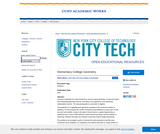Conditional Remix & Share Permitted
CC BY-NC-SA
Rating

This text is intended for a brief introductory course in plane geometry. It covers the topics from elementary geometry that are most likely to be required for more advanced mathematics courses. The only prerequisite is a semester of algebra.

The emphasis is on applying basic geometric principles to the numerical solution of problems. For this purpose the number of theorems and definitions is kept small. Proofs are short and intuitive, mostly in the style of those found in a typical trigonometry or precalculus text. There is little attempt to teach theorem-proving or formal methods of reasoning. However the topics are ordered so that they may be taught deductively.

The problems are arranged in pairs so that just the odd-numbered or just the even-numbered can be assigned. For assistance, the student may refer to a large number of completely worked-out examples. Most problems are presented in diagram form so that the difficulty of translating words into pictures is avoided. Many problems require the solution of algebraic equations in a geometric context. These are included to reinforce the student's algebraic and numerical skills, A few of the exercises involve the application of geometry to simple practical problems. These serve primarily to convince the student that what he or she is studying is useful. Historical notes are added where appropriate to give the student a greater appreciation of the subject.

This book is suitable for a course of about 45 semester hours. A shorter course may be devised by skipping proofs, avoiding the more complicated problems and omitting less crucial topics.

Subject:
Geometry
Material Type:
Textbook
Provider:
Provider Set:
New York City College of Technology
Author:
Henry Africk
01/01/2013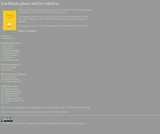Conditional Remix & Share Permitted
CC BY-SA
Rating

This book is designed for a semester-long course in Foundations of Geometry and meant to be rigorous, conservative, elementary and minimalistic.

Subject:
Geometry
Material Type:
Textbook
Author:
Anton Petrunin
08/13/2018Conditional Remix & Share Permitted
CC BY-NC
Rating

"Euclid's 'Elements' Redux" is an open textbook on mathematical logic and geometry based on Euclid's "Elements" for use in grades 7-12 and in undergraduate college courses on proof writing.

Many problem solvers throughout history wrestled with Euclid as part of their early education including Copernicus, Kepler, Galileo, Sir Isaac Newton, Ada Lovelace, Abraham Lincoln, Bertrand Russell, and Albert Einstein. This edition is part of an effort to ensure that tomorrow's great thinkers will have that same privilege.

Subject:
Geometry
Material Type:
Textbook
Author:
Daniel Callahan
11/18/2021Only Sharing Permitted
CC BY-NC-ND
Rating

LINEAR TRANSFORMATIONs ON THE PLAIN .
INTRODUCTION TO ISOHEDRALS , BASIC BLOCKS TO SYMMETRIC TILING.
TESSELLATIONs (PERIODIC & APERIODIC)
.
BRENIKOU , MACEDONIA EAST , HELLAS (GREECE).ARTIST (PAINTER & SCULPTOR) : EFSTRATOPOULOS NIKOLAOS ( efstrato71@gmail.com )MUSIC : Εύελδωρ - Λυρογηθής (ANCIEN...

Subject:
Architecture and Design
Computer Science
Graphic Arts
Geometry
Material Type:
Assessment
Homework/Assignment
Lecture Notes
Author:
GEORGAKAS NIKOLAOS (BRENIKOU)
10/26/2021Unrestricted Use
CC BY
Rating

The Module on Geometry starts by looking at the historical development of knowledge that the humankind gather along centuries and became later, about 300 BC, the mathematical subject called “Euclidian Geometry” because of the great work of Euclid. The inductive-deductive reasoning which characterizes this subject will be developed through investigation of your own conjectures on geometric objects and properties. You will explore geometry by using basic mechanical instruments (compass and straightedge) and computer software.

As you progress you will treat the Euclidian geometry using a referential system to locate points. The orthogonal Cartesian system of coordinates that you already know from secondary school is the most common referential system you will use in both two and three dimensions. You will also learn some other systems of coordinates that will empower you to do research in geometry and in other mathematical modules as well.

Going deeper in analyzing the axiomatic construction of Euclidean geometry you will learn new geometrical structures, generally designated as Non-Euclidian geometry. So, summarily speaking, this Module is about Euclidean geometry treated in both syntactical and analytical ways and encloses an introduction to Non-Euclidean Geometry, handled synthetically only.

Subject:
Geometry
Material Type:
Module
Provider:
African Virtual University
Provider Set:
OER@AVU
Author:
Marcos Cherinda
02/20/2018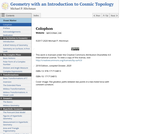Conditional Remix & Share Permitted
CC BY-SA
Rating

Motivated by questions in cosmology, the open-content text Geometry with an Introduction to Cosmic Topology uses Mobius transformations to develop hyperbolic, elliptic, and Euclidean geometry - three possibilities for the global geometry of the universe.

The text, written for students who have taken vector calculus, also explores the interplay between the shape of a space and the type of geometry it admits. Geometry is suitable for a semester course in non-Euclidean geometry or as a guide to independent study, with over 200 exercises and several essays on topics including the history of geometry, parallax and curvature, and research aimed at determining the shape of the universe.

Subject:
Geometry
Material Type:
Textbook
Author:
Michael P. Hitchman
11/18/2021Only Sharing Permitted
CC BY-NC-ND
Rating

This book presents Euclidean Geometry and was designed for a one-semester course preparing junior and senior level college students to teach high school Geometry. The book could also serve as a text for a junior level Introduction to Proofs course.

Subject:
Geometry
Material Type:
Textbook
Provider:
Ohio University Open Faculty Textbooks
Author:
Mark Barsamian
10/21/2020Conditional Remix & Share Permitted
CC BY-NC
Rating

Law of CosinesTMM 002 PRECALCULUS (Revised March 21, 2017)2c. Analyze general triangles. Routine analysis of side lengths and angle measurements using trigonometric ratios/functions, as well as other relationships.*Sample Tasks:The student can solve general triangles using trigonometric ratios and relationships including laws of sine and cosine.The student can compare similar triangles.The student can compute length and angle measurements inside complex drawings involving multiple geometric objects.The student can algebraically describe relationships inside complex drawings involving multiple geometric objects.

Subject:
Mathematics
Calculus
Geometry
Trigonometry
Material Type:
Module
Author:
Ohio Open Ed Collaborative
11/02/2020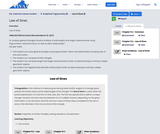Conditional Remix & Share Permitted
CC BY-NC
Rating

Law of SinesTMM 002 PRECALCULUS (Revised March 21, 2017)2c. Analyze general triangles. Routine analysis of side lengths and angle measurements using trigonometric ratios/functions, as well as other relationships.*Sample Tasks:The student can solve general triangles using trigonometric ratios and relationships including laws of sine and cosine.The student can compare similar triangles.The student can compute length and angle measurements inside complex drawings involving multiple geometric objects.The student can algebraically describe relationships inside complex drawings involving multiple geometric objects.

Subject:
Higher Education
Mathematics
Calculus
Geometry
Trigonometry
Material Type:
Module
Author:
Ohio Open Ed Collaborative
11/02/2020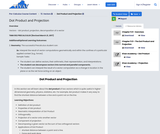Conditional Remix & Share Permitted
CC BY-NC
Rating

Vectors - dot product, projection, decomposition of a vectorTMM 002 PRECALCULUS (Revised March 21, 2017)AdditionalOptional Learning Outcomes:2. Geometry: The successful Precalculus student can:2e. Interpret the result of vector computations geometrically and within the confines of a particular applied context (e.g., forces).Sample Tasks:The student can define vectors, their arithmetic, their representation, and interpretations.The student can decompose vectors into normal and parallel components.The student can interpret the result of a vector computation as a change in location in the plane or as the net force acting on an object.

Subject:
Engineering
Higher Education
Mathematics
Geometry
Trigonometry
Material Type:
Module
Author:
Ohio Open Ed Collaborative
11/02/2020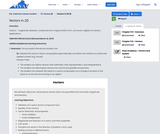Conditional Remix & Share Permitted
CC BY-NC
Rating

Vectors - magnitude, direction, component form, trigonometric form, unit vector, algebra of vectors, applications,TMM 002 PRECALCULUS (Revised March 21, 2017)AdditionalOptional Learning Outcomes:2. Geometry: The successful Precalculus student can:2e. Interpret the result of vector computations geometrically and within the confines of a particular applied context (e.g., forces).Sample Tasks:The student can define vectors, their arithmetic, their representation, and interpretations.The student can decompose vectors into normal and parallel components.The student can interpret the result of a vector computation as a change in location in the plane or as the net force acting on an object.

Subject:
Higher Education
Mathematics
Calculus
Geometry
Physics
Material Type:
Module
Author:
Ohio Open Ed Collaborative
11/02/2020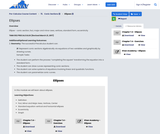Conditional Remix & Share Permitted
CC BY-NC
Rating

Ellipse - conic section, foci, major and minor axes, vertices, standard form, eccentricityTMM 002 PRECALCULUS (Revised March 21, 2017)AdditionalOptional Learning Outcomes:2. Geometry: The successful Precalculus student can:2f. Represent conic sections algebraically via equations of two variables and graphically by drawing curves.Sample Tasks:The student can perform the process “completing the square” transforming the equation into a standard form.The student can draw curves representing conic sections.The student can solve systems of equations involving linear and quadratic functions.The student can parametrize conic curves.

Subject:
Higher Education
Mathematics
Algebra
Geometry
Astronomy
Material Type:
Module
Author:
Ohio Open Ed Collaborative
11/02/2020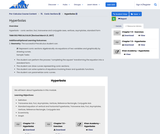Conditional Remix & Share Permitted
CC BY-NC
Rating

Hyperbola - conic section, foci, transverse and conjugate axes, vertices, asymptotes, standard formTMM 002 PRECALCULUS (Revised March 21, 2017)AdditionalOptional Learning Outcomes:2. Geometry: The successful Precalculus student can:2f. Represent conic sections algebraically via equations of two variables and graphically by drawing curves.Sample Tasks:The student can perform the process “completing the square” transforming the equation into a standard form.The student can draw curves representing conic sections.The student can solve systems of equations involving linear and quadratic functions.The student can parametrize conic curves.

Subject:
Higher Education
Mathematics
Geometry
Astronomy
Material Type:
Module
Author:
Ohio Open Ed Collaborative
11/02/2020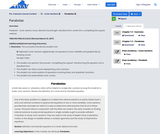Conditional Remix & Share Permitted
CC BY-NC
Rating

Parabola - conic section, focus, directrix, focal length, standard form, vertex form, completing the square method.TMM 002 PRECALCULUS (Revised March 21, 2017)AdditionalOptional Learning Outcomes:2. Geometry: The successful Precalculus student can:2f. Represent conic sections algebraically via equations of two variables and graphically by drawing curves.Sample Tasks:The student can perform the process “completing the square” transforming the equation into a standard form.The student can draw curves representing conic sections.The student can solve systems of equations involving linear and quadratic functions.The student can parametrize conic curves.

Subject:
Higher Education
Mathematics
Algebra
Geometry
Material Type:
Module
Author:
Ohio Open Ed Collaborative